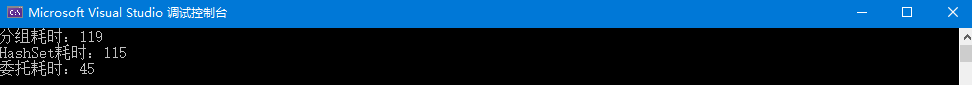# C# Linq 的三种去重方式（Distinct）

## 前言

``public class Person{    public string Name { get; set; }    public int Age { get; set; }}``

``            var list = new List<Person>();            for (int i = 0; i < 1000000; i++)            {                list.Add(new Person() { Age = 18, Name = "迷恋自留地" });            }            for (int i = 0; i < 1000; i++)            {                list.Add(new Person() { Age = 19, Name = "迷恋自留地" });            }``

## 第一种分组去重

``var  list1 = list.GroupBy(d => new { d.Age, d.Name })    .Select(d => d.FirstOrDefault())    .ToList();``

## 第二种 HashSet去重 （扩展方法）

C#中HashSet对于重复元素会进行过滤筛选，所以我们写下如下扩展方法（在静态函数中定义），遍历集合元素，最后利用HashSet进行过滤达到去重目的，如下：

``public static IEnumerable<TSource> Distinct<TSource, TKey>(        this IEnumerable<TSource> source,        Func<TSource, TKey> keySelector)    {        var hashSet = new HashSet<TKey>();        foreach (TSource element in source)        {            if (hashSet.Add(keySelector(element)))            {                yield return element;            }        }    }``

`` var  list2 = list.Distinct(d => new { d.Age, d.Name }).ToList();``

## 第三种 IEqualityComparer去重 （扩展方法）

``public static class Extensions{    public static IEnumerable<T> Distinct<T>(        this IEnumerable<T> source, Func<T, T, bool> comparer)        where T : class        => source.Distinct(new DynamicEqualityComparer<T>(comparer));    private sealed class DynamicEqualityComparer<T> : IEqualityComparer<T>        where T : class    {        private readonly Func<T, T, bool> _func;        public DynamicEqualityComparer(Func<T, T, bool> func)        {            _func = func;        }        public bool Equals(T x, T y) => _func(x, y);        public int GetHashCode(T obj) => 0;    }}``

``list = list.Distinct((a, b) => a.Age == b.Age && a.Name == b.Name).ToList();``

## 性能比较

``var list = new List<Person>();for (int i = 0; i < 1000000; i++){    list.Add(new Person() { Age = 18, Name = "jeffcky" });}var time1 = Time(() =>{    list.GroupBy(d => new { d.Age, d.Name })        .Select(d => d.FirstOrDefault())        .ToList();});Console.WriteLine(\$"分组耗时：{time1}");var time2 = Time(() =>{    list.Distinct(d => new { d.Age, d.Name }).ToList();});Console.WriteLine(\$"HashSet耗时：{time2}");var time3 = Time(() =>{    list.Distinct((a, b) => a.Age == b.Age && a.Name == b.Name).ToList();});Console.WriteLine(\$"委托耗时：{time3}");static long Time(Action action){    var stopwatch = new Stopwatch();    stopwatch.Start();    action();    stopwatch.Stop();    return stopwatch.ElapsedMilliseconds;}``https://www.cnblogs.com/netcore5/p/14776121.html